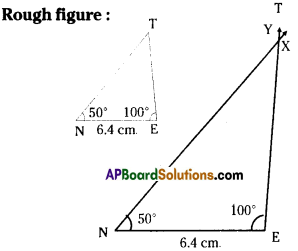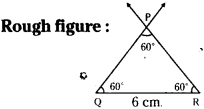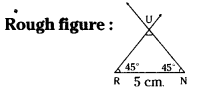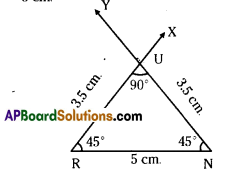# AP Board 7th Class Maths Solutions Chapter 9 Construction of Triangles Ex 3

AP State Syllabus AP Board 7th Class Maths Solutions Chapter 9 Construction of Triangles Ex 3 Textbook Questions and Answers.

## AP State Syllabus 7th Class Maths Solutions 9th Lesson Construction of Triangles Exercise 3Question 1.
Construct Δ NET with measurement NE = 6.4 cm, ∠N = 50° and ∠E = 100°.
Solution:
NE = 6.4 cm, ∠N = 50° and ∠E = 100°Step -1: Draw a rough sketch of a triangle and label it with the given measurements.
Step -2: Draw a Line segment NE of length 6.4 cm.
Step -3: Draw a ray $$\overrightarrow{\mathrm{NX}}$$ maktng an angle 50° at N.
Step -4: Draw a ray $$\overrightarrow{\mathrm{EY}}$$ making an angle 100° at E. Extend the ray $$\overrightarrow{\mathrm{NX}}$$ if necessary to intersect ray $$\overrightarrow{\mathrm{EY}}$$
Step – 5: Mark the intersecting point of the two rays as T.
We have the required ΔNET.Question 2.
Construct ΔPQR such that QR =6 cm, ∠Q = ∠R = 60°. Measure the other two sides of the triangle and name the triangle.
Solution:QR = 6cm ∠Q = ∠R = 60°.
Step -1: Draw a rough sketch of a triangle and label it with the given measurements.
Step -2: Draw a line segment QR of length 6 cm.
Step -3: Draw a ray $$\overrightarrow{\mathrm{QX}}$$ making an angle 60° at Q
Step -4: Draw a ray $$\overrightarrow{\mathrm{RY}}$$ making an angle 60° at R.
Step -5: Mark the intersecting point of the two rays as P. PQ = 6cm and PR = 6 cm
∴ The triangle is an equilateral triangle.Question 3.
Construct ΔRUN in which RN = 5cm, ∠R = ∠N = 45°. Measure the other angle and other sides. Name the triangle.
Solution:
RN = 5cm, ∠R = ∠N = 45°.Step -1: Draw a rough sketch of a triangle and label it with the given measurements.
Step -2: Draw a line segment RN of length 5cm.
Step -3: Draw a ray $$\overrightarrow{\mathrm{RX}}$$ making an angle 45° at R.
Step -4: Draw a ray $$\overrightarrow{\mathrm{NY}}$$ making an angle 45° at N.
Step -5: Mark the intersecting point of the two rays as U. ΔRUN is the required triangle
∠U = 90°, RU = UN = 3.5 cm.
∴ The triangle is an isosceles right angled triangle.